Next: Exponentials, ex Up: Algebraic numbers Previous: Algebraic numbers

### Average number of different roots

The probability of a random polynomial (mod n) of degree k having i roots is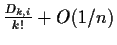. Dk,i are called the rencontre numbers. Dk,1/k! is the limiting value when n grows of the number of polynomials which will have a single root, and hence suitable for computing signatures. A description of these numbers can be found in  pp. 65. The exponential generating function for them is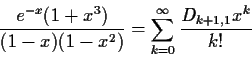The limiting value, when the degree of the polynomial grows, is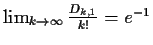. In other words, for random polynomials of high degree and for large size fields, 36.79% of the time these polynomials will have a single root suitable for use as a signature.

If we have more than one linear factor for all the values of n that we are prepared to try (notice that for polynomials of degree 2 we either have two linear factors or none), then we have multiple choices. Whenever we have multiple choices we have to compute all signatures arising from them. So if we have a square root and an algebraic number which factors in no less than 3 linear terms we will have to compute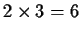signatures.

If all the signatures arethe expression is not zero. If all Sn(e)=0 then the expression is identically zero with some probability of error. Else we cannot decide and the procedure fails.

Example. Let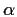and p(x) be defined as before. The following is a standard normalization of a rational expression containing algebraic numbers: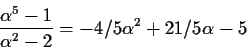To compute the signature of these expressions, we need to find an n for which p(x) factors with only one linear factor. n=5 will fail to compute some fractions on the right hand side. For n=7, p(x) does not factor. For n=11,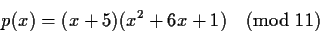has a single linear factor and we decide to use it. So we set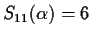and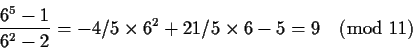as expected.Next: Exponentials, ex Up: Algebraic numbers Previous: Algebraic numbers
Gaston Gonnet
1999-07-04